top of page
Search

# PATTERN RECOGNITION

Updated: Aug 6, 2021

Author- Simreeta Saha, Rupesh Kumar

Pattern detection is a part of machine learning where we generally use a classification model with the formation of a confusion matrix. But the major issue in this part is the classification of unbalanced data. So, when such errors must also be considered, then the Matthews Correlation Coefficient arises as the best choice. Finally, a set of null-biased multi-perspective Class Balance Metrics is proposed which extends the concept of Class Balance Accuracy to other performance metrics.Figure 1: Balanced dataset for heat images Methodology

## Confusion Matrix

A confusion matrix is a table that is often used to describe the performance of a classification model on a set of test data for which the values are known. Consider a dataset

D={ d1 , d2 , d3 ,…….. dn}Now taking DOT(𑁦) product with ƛ, we get:This confusion matrix (CM) can be written as:In this matrix, if we put C=2, we get,Now putting 1 as Positive (P) and 2 as Negative (N), we get,• Mpp - True positive

• Mpn - True negative

• Mnp - False positive

• Mnn - False negative## Metrics based on binary confusion matrix:Figure 2 : Confusion matrix for binary classification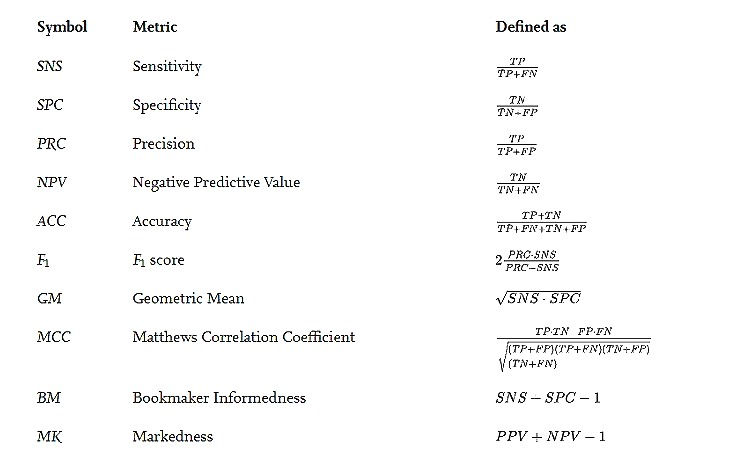Figure 3 : Definition of classification performance metrics.

## Defining class imbalance

The concept of class imbalance is relatively clear: it arises when the dataset has a different number of elements in positive and negative classes. However, its formalization is far from being univocally accepted. For instance, the imbalance is characterized by the dominance (Dom) or prevalence relationship between the positive class and the negative class and is defined as:

TPR−TNR

. This value is later employed to compensate performance metrics affected by the imbalance problem. However, the dominance is not exactly a measure of the imbalance in the dataset because it considers imbalance in the outcomes of the classifier.Figure 2 : Classification performance metrics as a function of imbalance.

## Performance metric bias function

Bias depends on three variables, i.e,

`                       Bμ = Bμ(λPP, λNN, δ). `

The approach for their representation is based on the heat volumes.Figure 5 : Heat volumes of bias for each performance metric

Performance metrics like MCCn have low bias values for many points in the (λPP, λNN, δ) space, and for those, the expressive power of the whole range of colours is not completely exploited. So it is a good approach to select the colour of each point not directly based on bias, but on the relative value of bias within the range of values for their corresponding metric. The colour-map is rescaled to show the relative bias with the value −1 to +1. The result can be depicted as: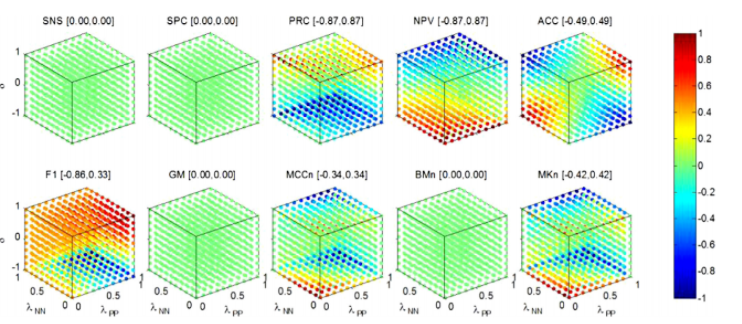Figure 6 : Heat volumes of relative bias for each performance metric

We can also represent as a set of heat maps. In the figure, each heat map corresponds to the metric bias for a fixed value of the imbalance

Bμ = Bμ (λPP, λNN, δ0).

The heat map is shown as:If δ = δ0 = 0.95. Therefore Bμ = Bμ (λPP, λNN, δ0) depends on only two variables (λPP and λNN) and can be represented as a heat map as:Figure 7: Heat maps of bias for each performance metric (δ = 0.95)

The above information can also be presented in the form of contour graphs where the colours represent the absolute value of bias.Figure 8 : Contour graphs of bias for each performance metric (δ = 0.95)Figure 9 : Functions and single-valued indicators assessing bias in performance metrics due to class imbalance

## Bias indicators depending on δ

The results obtained for each bias indicator and performance metric σ Bμ(δ) are summarized, where unbiased performance metrics (SNS, SPC, GM, BMn) have been omitted.Figure 10 : Bias indicators for singular classifiers: σ Bμ(δ)

There are four types of Non-null indicators. Their dependence on δ is plotted as :Figure 11 : Bias indicators for singular classifiers

There’s an alternate way too where we can assume that λPP and λNN are randomly and uniformly distributed across the [0, 1] range and the bias Bμ(δ) can then be statistically characterized. The probability density function (pdf[Bμ(δ)]) is derived for each performance metric and can be represented as: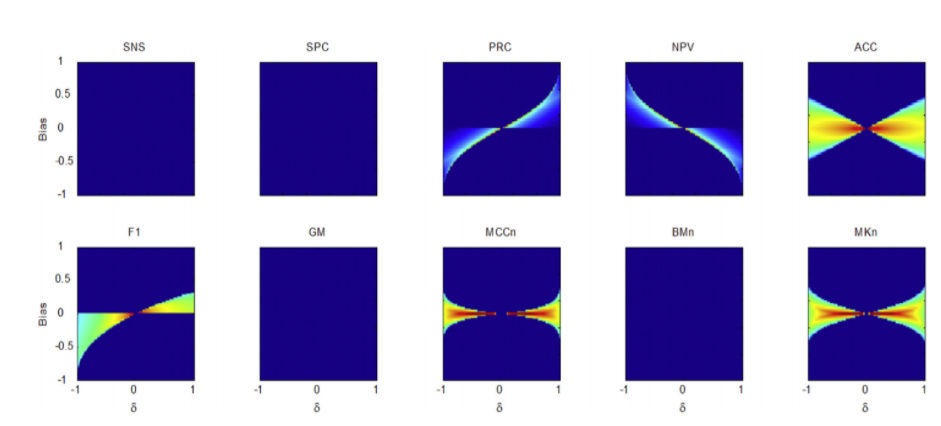Figure 12 : Probability density function pdf[Bμ(δ)] for each performance metric

Statistical indicators are generically denoted as ψBμ(δ). The results obtained for each performance metric are depicted below. Unbiased performance metrics (SNS, SPC, GM, BMn) have been omitted for convenience. NPV presents symmetric behaviour to PRC so it has also been omitted.Figure 13 : Local statistical indicators of bias ψBμ(δ) for each performance metric

## Single-valued bias indicators

There are different ways to obtain single-valued bias indicators. One of them is to consider bias functions σ Bμ(δ) as summarized assuming that δ is randomly and uniformly distributed within the range [−1, 1], the mean values of each measure can be computed. The results for each performance metric are shown asTable 14 : Mean values of bias functions for singular classifiers σ Bμ(δ)

Applying the same method on bias functions ψBμ(δ), mean values of these measures can also be obtained. Results for each metric are shown asTable 15 : Mean values of bias of local statistical indicators ψBμ(δ)

Single-valued bias indicators can also be obtained by focusing on their value for extremely imbalanced datasets. It can be done by considering the bias metrics of singular classifiers (σ Bμ(δ)), their results are shown asTable 16 : Bias indicators on singular classifiers (σ Bμ(δ)) for extremely imbalanced datasets

The extremely imbalanced case for local statistical indicators (ψBμ(δ)), is shown in Table below:Table 17 : Bias measures on local statistical indicators (ψBμ(δ)) for extremely imbalanced datasets

Let us suppose that λPP and λNN are randomly and uniformly distributed across the range[0, 1] while δ lies within the range [−1, 1], and Bμ is statistically characterized. Probability density function (pdf(Bμ)) is derived for each performance metric except NPV because it shows symmetric behaviour to PRC and can be represented asFigure 18 : Probability density function pdf(Bμ) for each performance metric

The results obtained for each performance metric on global statistical indicators which are denoted as ψBμ can be represented as: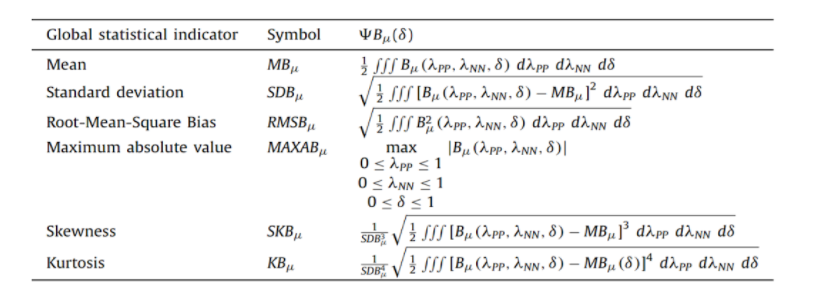Table 19 : Global statistical indicators on bias (ψBμ(δ))Table 20: Global statistical indicators of bias ψBμ for each performance metric.

• Single-valued bias indicators can also be presented in a graphical form as shown below:Figure 21 : Single-valued bias indicators for each performance metric

## Symmetry of Bias Functions

It is important to study the symmetry of bias function in order to categorize them and for that we need Matthews Correlation Coefficient (MCC). The imbalance coefficient δ = 0.95.Figure 22 : Study of symmetry for BMCC(λNN, λPP) with δ = 0.95

In the first step, an anti-clockwise 90° rotation on the (λPP, λNN) plane is performed as shown in the upper right-hand-side plot. In the second step again anti-clockwise 90º rotation is done and is shown in the lower right-hand-side graph. Then the sign of the bias values is changed that can be seen in the lower left-hand-side plot. We can observe that the result coincides with the original heat map. Therefore the symmetry in mathematical terms can be written as:

### BMCC (λPP, λNN, δ) = −BMCC (^PP,^NN, δ) = −BMCC (1 − λPP, 1 − λNN, δ)

MCC bias function shows an order-2 (180º) rotational odd symmetry on the original axes plane (λPP, λNN). The bias function shows dual behaviour which is called Type I symmetry which can be understood using the following notation.

If the sign of δ is inverted, bias function shows symmetry with the principal diagonal on the (λPP, λNN) plane as:

## BMCC (λNN, λPP, −δ) = BMCC (λPP, λNN, δ)

If the bias of precision is considered then no symmetry on the (λPP, λNN) plane can be found But it exhibits a symmetry in the (λPP, λNN, δ) space where heat volume δ = 0.95 is represented in the upper left-hand-side plot as can be observed in the figure given belowFigure 23 : Study of symmetry for BPRC(λNN, λPP, δ) with δ = 0.95

In the first step, a mirror symmetry with δ = 0 plane is performed and the result is shown in the upper right-hand-side plot. Then in the second step, the sign of the bias values is changed and the results are shown in the lower right-hand-side plot. In the final step a second mirror symmetry is performed with respect to the anti-diagonal plane drawn in the third plot which is shown in the lower left-hand-side plot. It can be observed that the result is similar to the original heat volume. The above can be termed as double mirror symmetry which enables the bias to be defined on a new set of axes (^PP, ^NN, delta ). They are related to the original set through the expressions ^PP = 1 − λNN; ^NN = 1 − λPP; delta= −δ. The above can be written in mathematical terms as:

```BPRC (λPP, λNN, δ) = −BPRC (^PP,^NN,delta)
= −BPRC (1 − λNN, 1 − λPP, −δ)```

Hence we can say that the precision (PRC) bias function shows a double mirror odd symmetry which is also referred to as anti-symmetry in the (λPP, λNN, δ) space. This behaviour is called Type II symmetry. Except F1 score bias function for every metric exhibits Conclusion this type of symmetry.

## Clustering Performance Metrics Based on their Bias

The performance metrics can be grouped into several clusters. To perform clustering, the 39 single-valued bias indicators are considered. Each performance metric is to be featured by a point in an 39*1 shape. The approach A as illustrated in the figure involves the selection of 2 highly significant bias indicators and the projection of the points on that plane. RMSBμ is an indicator of the mean global bias, rmsBεP μ is the mean gauge of bias. It can be seen that 3 or 4 clusters could be formed. But if we go in more depth then it shows that PRC and NPV have symmetric behaviour for many bias indicators. They have appeared together in the A because the selected indicators compute squared mean, hiding their symmetric characteristics.Figure 24 : Bidimensional representation of performance metrics according to their bias indicators

To overcome this problem, dimensionality reduction can be made by selecting a different pair of bias indicators. In fig B, MAXAB is a global indicator of the absolute maximum value of bias but stills it hides the symmetry and mBεP μ is mean gauge of bias for extremely positive imbalanced datasets that reveals the symmetry. From this we can clearly see 5 clusters.

Above these an alternative approach is the arbitrary and reductionist selection that involves the consideration of the full set of indicators and the performance of bidimensional reduction. Principal Component Analysis (PCA) in C, and Multidimensional Scaling (MDS) in D, are employed as the techniques for this reduction.

## Clustering InformationFigure 25 : Clusters of performance metrics attending to their bias

We can also represent grouping of performance metrics according to the bias behaviour using a dendrogram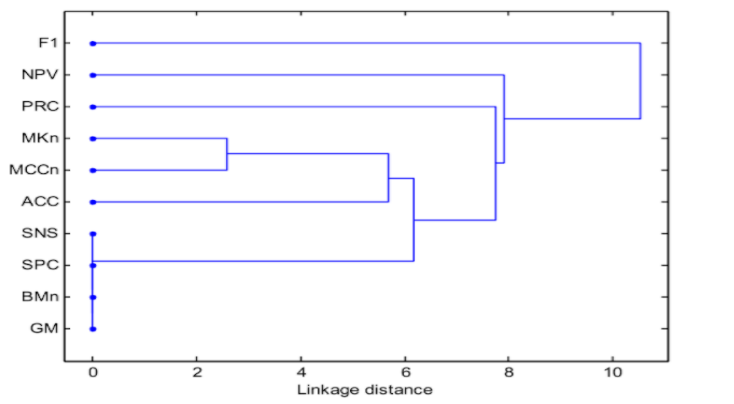Figure 26 : Dendrogram of performance metrics according to their bias measures

## Conclusions

This presents an extensive and systematic study of the impact of class imbalance on classification performance metrics. Imbalance Coefficient has been defined for characterizing the disparity between classes, which is used to surpass the Imbalance Ratio. This derives several practical procedures to determine the bias’s quantitative value of a metric.

This develops a guide to select performance metrics in the presence of imbalance classes. Different clusters of performance metrics have been identified that involve the use of Geometric Mean or Bookmaker Informedness as the best null-biased metrics. There may be a chance of classification errors for that solution, the Matthews Correlation Coefficient is used. A set of null-biased multi-perspective Class Balance Metrics is also proposed which helps to understand the concept of Class Balance Accuracy.

## Github:

• https://github.com/Simreeta/Class_imbalance_binary_confusion_matrix

## References:

• www.dataschool.io

• https://www.sciencedirect.com/science/article/pii/S0031320319300950

• V. López, A. Fernández, S. García, V. Palade, F. Herrera

• An insight into classification with imbalanced data: empirical results and current trends on using data intrinsic characteristics Inf. Sci., 250 (2013), pp. 113-141

• V. García, R.A. Mollineda, J.S. Sánchez

• Index of balanced accuracy: a performance measure for skewed class distributions

• Iberian Conference on Pattern Recognition and Image Analysis, Berlin, Heidelberg, Springer (2009, June), pp. 441-448